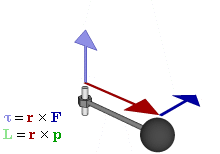## FANDOM

162 PagesAnimation showing the relationship between force (F), torque (τ), linear momentum (p), angular momentum (L), and position (r) of arotating object.

Torque (τ) is a measure of how much a force causes an object to rotate around a pivot point. The SI unit for torque is the Newton metre (N·m). Torque is a pseudovector, since it can either be clockwise or counterclockwise. The direction of the vector will be perpendicular to the axis of rotation as directed by the right-hand rule. The formula for torque is

$\tau = r \times F$

F is equal to the applied force and r is the position vector representing the distance between the point the force is applied and the pivot point.

Often, the direction of torque is not necessary; only the magnitude is required. In this case, if |F| is the magnitude of the force applied and d is the distance from the pivot point,

$\| \tau \| = \| F \| d$
Community content is available under CC-BY-SA unless otherwise noted.